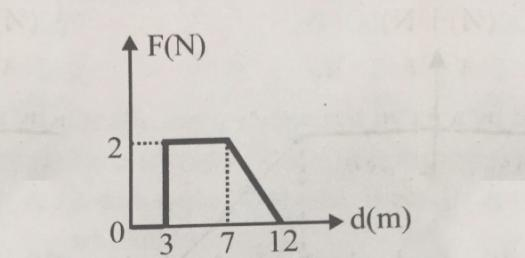# Physics Quiz: Distance, Force And Displacement

8 Questions | Total Attempts: 1799SettingsCreate your own Quiz.

• 1.
How is speed calculated?
• A.

Speed= distance ÷ time

• B.

Speed= area

• C.

Speed= distance × time

• 2.
Which one of these graphs are showing fast movement?
• A.
• B.
• C.
• 3.
Velocity calculated by
• A.

Displacement ÷ time

• B.

Distance +Time

• C.

Time ÷ Displacement

• 4.
Which of these are forces?
• A.

Displacement

• B.

Wind

• C.

Upthrust

• D.

Slide

• 5.
Weight is a force
• A.

True

• B.

False

• 6.
Mass is measured in what unit?
• 7.
A Moment= force × -------- from the ---- (enter the two missing words separated by a comma)
• 8.
When Forces are not balanced, the object is not moving.
• A.

True

• B.

False

Related TopicsBack to top
×

Wait!
Here's an interesting quiz for you.